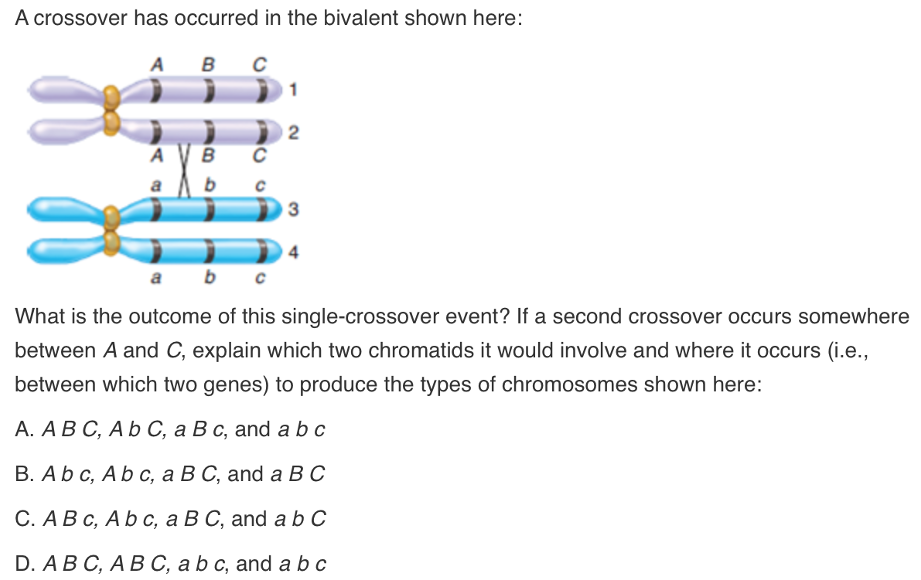# Problem: A crossover has occurred in the bivalent shown below:What is the outcome of this single-crossover event? If a second crossover occurs somewhere between A and C, explain which two chromatids it would involve and where it occurs (i.e. between which two genes to produce the types of chromosomes shown here:A. A B C, A b C, a B c, and a b cB. A b c, A b c, a B C, and a B CC. A B c, A b c, a B C, and a b CD. A B C, A B C, a b c, and a b c

###### FREE Expert Solution

During meiosis, chromosomes that are duplicated homologs pair up and crossover. Crossover is the exchange of genetic material between these homologs and result in recombinant chromosomes. This happens as matching regions of matched chromosomes break and reconnect to the other chromosome. At least one crossover per chromosome should occur so that the homologous pair stay together until metaphase I.###### Problem Details

A crossover has occurred in the bivalent shown below:

What is the outcome of this single-crossover event?

If a second crossover occurs somewhere between A and C, explain which two chromatids it would involve and where it occurs (i.e. between which two genes to produce the types of chromosomes shown here:

A. A B C, A b C, a B c, and a b c

B. A b c, A b c, a B C, and a B C

C. A B c, A b c, a B C, and a b C

D. A B C, A B C, a b c, and a b c